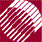ECE532 Biomedical Optics © 1998 Steven L. Jacques, Scott A. Prahl Oregon Graduate Institute

Launching photons

The programming that launches and propagates each photon through the tissue is enclosed within a DO-WHILE LOOP which launches N photons (N = Nphotons).

/**** RUN
Launch N photons, initializing each one before progation.
*****/
do {

/* PROGRAM SITS WITHIN THIS DO-WHILE LOOP THAT LAUNCHES N PHOTONS */

/* If photon dead, then launch new photon. */
} /* end RUN */
while (i_photon < Nphotons);

On each launch of a new photon the following is done. The photon counter (i_photon) is incremented. The photon weight (W) is set to value 1. The photon status (photon_status) is set to "ALIVE". Later when the program determines the photon should be terminated, the photon_status will be set to "DEAD".

Each "photon" is launched at a position (x, y, z) which is set to the origin (0, 0, 0). The initial trajectory of the photons is described by the the trajectory vector (ux, uy, uz) which cites the projection of the trajectory onto the x, y, and z coordinates. In spherical coordinates, the trajectory is described by the deflection angle off the z-axis (theta) and by the azimuthal angle around the z-axis (psi). For an isotropic point source the initial theta value is randomly set by selecting a value for cosine(theta), called costheta, between -1 and 1 (corresponding to 180° - 0° with respect to the z-axis). The initial psi value and is randomly set between 0 and 2*PI. The term sintheta is a temporary variable related to costheta. The values costheta, sintheta, and psi are used to project the trajectory vector onto the x, y, and z axes to yield the values ux, uy, and uz. The trajectory vector (ux, uy, uz) has a unit length.

/**** LAUNCH
Initialize photon position and trajectory.
Implements an isotropic point source.
*****/
i_photon += 1;	/* increment photon count */
W = 1.0;                    /* set photon weight to one */
photon_status = ALIVE;      /* Launch an ALIVE photon */

x = 0;                      /* Set photon position to origin. */
y = 0;
z = 0;

/* Randomly set photon trajectory to yield an isotropic source. */
costheta = 2.0*RandomNum - 1.0;
sintheta = sqrt(1.0 - costheta*costheta);	/* sintheta is always positive */
psi = 2.0*PI*RandomNum;
ux = sintheta*cos(psi);
uy = sintheta*sin(psi);
uz = costheta;

to next page | Chapter 4 | Course | Home

©1998, Steven L. Jacques, Scott A. Prahl, Oregon Graduate Institute, Oregon Medical Laser Center